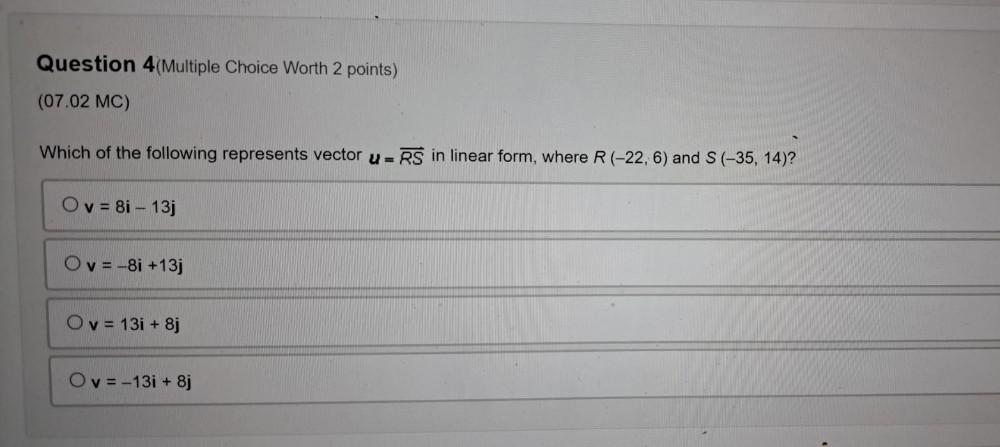Question:

# Which of the following represents vector u=RS in linear form, where R (-22, 6) and S(-35, 14)? O v= 8i - 13j O v= -8i +13j O v = 131 + 8j O v= -13i + 8jWhich of the following represents vector u=RS in linear form, where R (-22, 6) and S(-35, 14)? O v= 8i - 13j O v= -8i +13j O v = 131 + 8j O v= -13i + 8j